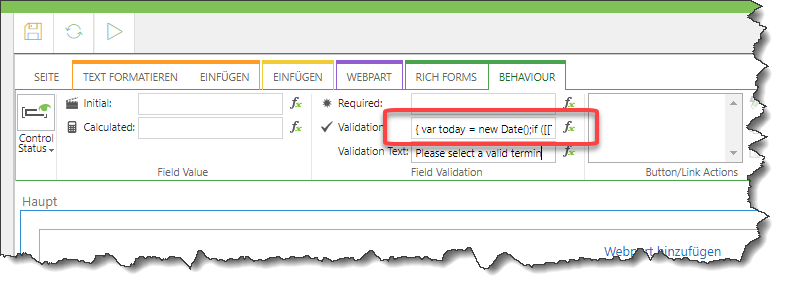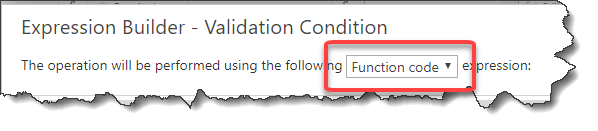## Search

•any suggestion?

•You could apply a custom-validation to the field "Teknik Bölüm Termin Talep". This would trigger the field-value to be invalid and an according text will be displayed.

•Hello Henning,

Thank's so much for your reply but I do not know how to get the current_date for custom validation. I need a syntax that gives me the current_date THEN I can compare. So my example as above.

Let's say If my user edits the form today so today is 6/1/2019  and the "Teknik bolum1.Termin" date is : 21/1/2020

My user can not select from(LOV) value as  "Evet 2.Termin"  because "Tekink Bolum 2.Termin" date is is 21/3/2020

and too earlier then TODAY.

Actually my user must not select LOV values IF the current date early then my calculated dates as below fields

Teknik Bolum 1.Termin

Teknik Bolum 2.Termin

Teknik Bolum 3.Termin

That's why I need a custom field for my Rich Form and calculates and sets the current date on every editing operation

Then I will compare with my 3 date fields. BUT How :-)

•Well, you will need to open the expression builder for the validationand write something like this:

``var today = new Date();if ([[Teknik Bölüm Termin Talep]] == "Evet 1.Termin" && [[Teknik Bolum 1.Termin]] < today)  return false;if ([[Teknik Bölüm Termin Talep]] == "Evet 2.Termin" && [[Teknik Bolum 2.Termin]] < today)  return false;if ([[Teknik Bölüm Termin Talep]] == "Evet 3.Termin" && [[Teknik Bolum 3.Termin]] < today)  return false;return true;``

Make sure, that you set the expression to "Function code"•Hello Henning;

var today = new Date();
if ([[Teknik_x0020_B_x00f6_l_x00fc_m_x4]] == "Evet 1.Termin" || [[Teknik_x0020_B_x00f6_l_x00fc_m_x0]] < today)
return false;
if ([[Teknik_x0020_B_x00f6_l_x00fc_m_x4]] == "Evet 2.Termin" || [[Teknik_x0020_B_x00f6_l_x00fc_m_x2]] < today)
return false;
if ([[Teknik_x0020_B_x00f6_l_x00fc_m_x4]] == "Evet 3.Termin" || [[Teknik_x0020_B_x00f6_l_x00fc_m_x3]] < today)
return false;
return true;

Then it works:-))